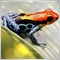# Problems when customizing an ADX indicator57

Hello,

I'm trying to build a modification of the ADX. I am only interested in the values of D+ and D-. In order to improve the visibility of a signal, as soon as one of the values is higher than the other and additionally a threshold is exceeded, a color change should happen.

I seem to have a problem with the ADXBuffer, but I don't understand which one yet.

```//+------------------------------------------------------------------+
//+------------------------------------------------------------------+

#property indicator_separate_window
#property indicator_buffers 4
#property indicator_plots   1
//+------------------------------------------------------------------+
input int                  InpMAPeriod       =           14;    // MA period
input ENUM_APPLIED_VOLUME  InpAppliedVolume  =  VOLUME_TICK;    // Volumes
input int                  inpHistWidth      =            4;    // Histogramwidth
input color                inpColor          =clrDodgerBlue;    // Color
input color                inpColorUP        =      clrLime;    // Color UP
input color                inpColorDN        =       clrRed;    // Color DN
input color                inpColorOD        =      clrGray;
input double               Threshold         =           40;
//+------------------------------------------------------------------+
int      inpLineWidth = 1;
int      MAHandle;
double   DI_plusBuffer[];
double   DI_minusBuffer[];
//+------------------------------------------------------------------+
//| Custom indicator initialization function                         |
//+------------------------------------------------------------------+
int OnInit()
{
SetIndexBuffer(1,DI_plusBuffer,INDICATOR_CALCULATIONS);
SetIndexBuffer(2,DI_minusBuffer,INDICATOR_CALCULATIONS);

PlotIndexSetInteger(0,PLOT_DRAW_BEGIN,InpMAPeriod);                        // sets first bar from what index will be drawn
PlotIndexSetInteger(0,PLOT_LINE_WIDTH,inpLineWidth);
PlotIndexSetInteger(0,PLOT_DRAW_TYPE,DRAW_COLOR_HISTOGRAM);
PlotIndexSetInteger(0,PLOT_LINE_WIDTH,inpHistWidth);
PlotIndexSetInteger(0,PLOT_COLOR_INDEXES,3);
PlotIndexSetInteger(0,PLOT_LINE_COLOR,0,inpColorUP);
PlotIndexSetInteger(0,PLOT_LINE_COLOR,1,inpColorDN);
PlotIndexSetInteger(0,PLOT_LINE_COLOR,2,inpColorOD);

IndicatorSetString(INDICATOR_SHORTNAME,"ADX Hist("+string(InpMAPeriod)+")");  // name for DataWindow and indicator subwindow label

// get MA handle
if(MAHandle==INVALID_HANDLE)                                               // check MA handle
{
return(INIT_FAILED);
}
return(INIT_SUCCEEDED);
}
//+------------------------------------------------------------------+
//| Force Index                                                      |
//+------------------------------------------------------------------+
int OnCalculate(const int rates_total,
const int prev_calculated,
const int begin,
const double &price[])
{
int i,start,to_copy;

if(rates_total<InpMAPeriod)               // check for rates total
return(0);                             // try again next tick

if(BarsCalculated(MAHandle)<rates_total)  // check for MA ready
return(0);                             // try again next tick
// calculate amount of data needed
to_copy=(prev_calculated==0)?rates_total:rates_total-prev_calculated+1;

//   if(CopyBuffer(MAHandle,0,0,to_copy,ADXBuffer)!=to_copy)   // get ma buffer
//      return(0);
if(CopyBuffer(MAHandle,1,0,to_copy,DI_plusBuffer)!=to_copy)   // get ma buffer
return(0);
if(CopyBuffer(MAHandle,2,0,to_copy,DI_minusBuffer)!=to_copy)   // get ma buffer
return(0);
// preliminary calculations
start=(prev_calculated<InpMAPeriod)?InpMAPeriod:prev_calculated-1;

if(InpAppliedVolume==VOLUME_TICK)         // the main loop of calculations
{
for(i=start; i<rates_total && !IsStopped(); i++)
{
if(DI_plusBuffer[i] > DI_minusBuffer[i]){
if(DI_plusBuffer[i] > Threshold){
}else{
}
Print("DI_plusBuffer["+i+"]*"+DI_plusBuffer[i]+"*");
}else{
if(DI_minusBuffer[i] > Threshold){
}else{
}
Print("DI_minusBuffer["+i+"]*"+DI_minusBuffer[i]+"*");
}
}
}
else
{
for(i=start; i<rates_total && !IsStopped(); i++)
{
if(DI_plusBuffer[i] > DI_minusBuffer[i]){
if(DI_plusBuffer[i] > Threshold){
}else{
}
Print("DI_plusBuffer["+i+"]*"+DI_plusBuffer[i]+"*");
}else{
if(DI_minusBuffer[i] > Threshold){
}else{
}
Print("DI_minusBuffer["+i+"]*"+DI_minusBuffer[i]+"*");
}
}
}
return(rates_total);
}```

In the log I can see that the ADXBuffer gets the correct values. However, nothing is plotted at all in the indicator itself.

Thanks,

h4rry19998

h4rry: However, nothing is plotted at all in the indicator itself.

You have plots=1 and only ADX is indicator Data. Stop what you are doing and go back to the original code.

Change plots to two, make the first two buffers (DX±) indicator data. Make ADX the third buffer. Four lines are changed, NO OTHER CODE needs to be changed.57

Hi William,

thanks for the answer. Did you mean like that?

`#property indicator_plots   2`

and

```   SetIndexBuffer(0,DI_plusBuffer,INDICATOR_DATA);
SetIndexBuffer(1,DI_minusBuffer,INDICATOR_DATA);

or

```   SetIndexBuffer(0,DI_plusBuffer,INDICATOR_DATA);
SetIndexBuffer(1,DI_minusBuffer,INDICATOR_DATA);

But it doesn't matter. I tested it both ways, but I get the same result. Nothing gets plotted.57

i could fix it

```//+------------------------------------------------------------------+
//+------------------------------------------------------------------+

#property indicator_separate_window
#property indicator_buffers 4
#property indicator_plots   2
//+------------------------------------------------------------------+
input int                  InpMAPeriod       =           14;    // MA period
input ENUM_APPLIED_VOLUME  InpAppliedVolume  =  VOLUME_TICK;    // Volumes
input int                  inpHistWidth      =            4;    // Histogramwidth
input color                inpColor          =clrDodgerBlue;    // Color
input color                inpColorUP        =      clrLime;    // Color UP
input color                inpColorDN        =       clrRed;    // Color DN
input color                inpColorOD        =      clrGray;
input double               Threshold         =           40;
//+------------------------------------------------------------------+
int      inpLineWidth = 1;
int      MAHandle;
double   DI_plusBuffer[];
double   DI_minusBuffer[];
//+------------------------------------------------------------------+
//| Custom indicator initialization function                         |
//+------------------------------------------------------------------+
int OnInit()
{
// indicator buffers mapping
SetIndexBuffer(2,DI_plusBuffer,INDICATOR_CALCULATIONS);
SetIndexBuffer(3,DI_minusBuffer,INDICATOR_CALCULATIONS);

PlotIndexSetInteger(0,PLOT_DRAW_BEGIN,InpMAPeriod);                        // sets first bar from what index will be drawn
PlotIndexSetInteger(0,PLOT_LINE_WIDTH,inpLineWidth);
PlotIndexSetInteger(0,PLOT_DRAW_TYPE,DRAW_COLOR_HISTOGRAM);
PlotIndexSetInteger(0,PLOT_LINE_WIDTH,inpHistWidth);
PlotIndexSetInteger(0,PLOT_COLOR_INDEXES,3);
PlotIndexSetInteger(0,PLOT_LINE_COLOR,0,inpColorUP);
PlotIndexSetInteger(0,PLOT_LINE_COLOR,1,inpColorDN);
PlotIndexSetInteger(0,PLOT_LINE_COLOR,2,inpColorOD);

IndicatorSetString(INDICATOR_SHORTNAME,"ADX Hist("+string(InpMAPeriod)+")");  // name for DataWindow and indicator subwindow label

// get MA handle
if(MAHandle==INVALID_HANDLE)                                               // check MA handle
{
return(INIT_FAILED);
}
return(INIT_SUCCEEDED);
}
//+------------------------------------------------------------------+
//| Force Index                                                      |
//+------------------------------------------------------------------+
int OnCalculate(const int rates_total,
const int prev_calculated,
const int begin,
const double &price[])
{
int i,start,to_copy;

if(rates_total<InpMAPeriod)               // check for rates total
return(0);                             // try again next tick

if(BarsCalculated(MAHandle)<rates_total)  // check for MA ready
return(0);                             // try again next tick
// calculate amount of data needed
to_copy=(prev_calculated==0)?rates_total:rates_total-prev_calculated+1;

if(CopyBuffer(MAHandle,1,0,to_copy,DI_plusBuffer)!=to_copy)   // get ma buffer
return(0);
if(CopyBuffer(MAHandle,2,0,to_copy,DI_minusBuffer)!=to_copy)   // get ma buffer
return(0);
// preliminary calculations
start=(prev_calculated<InpMAPeriod)?InpMAPeriod:prev_calculated-1;

if(InpAppliedVolume==VOLUME_TICK)         // the main loop of calculations
{
for(i=start; i<rates_total && !IsStopped(); i++)
{
if(DI_plusBuffer[i] > DI_minusBuffer[i]){
}else{
}
}else{
}else{
}
}
}
}
else
{
for(i=start; i<rates_total && !IsStopped(); i++)
{
if(DI_plusBuffer[i] > DI_minusBuffer[i]){
}else{
}
}else{